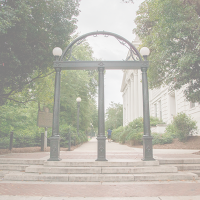# Number Theory Seminar: Pete L. Clark (UGA)

Date and time:
Boyd Room 304

Title of talk: Acyclotomy of torsion on elliptic curves

Abstract: Much past and contemporary work studies rational points on modular curves defined over number fields, i.e., finite degree extensions of Q. But what about algebraic extensions of infinite degree? Two old results provide inspiration: if A/F is an abelian variety over a number field, Ribet showed that A has finite torsion over the maximal cyclotomic extension of F and Zarhin determined necessary and sufficient conditions for A to have finite torsion over the maximal abelian extension of F.

In view of Ribet's Theorem, for any elliptic curve E defined over the maximal abelian extension of Q, the torsion subgroup is finite. So it is natural to ask: is there a uniform bound? Michael Chou has shown that the answer is yes if we restrict to elliptic curves extended from Q. I showed the answer is yes if we restrict to elliptic curves with complex multiplication. In this talk I will discuss these results, some refinements and some related open questions.

###SpeakerPete L. Clark

Professor
plclark@gmail.com